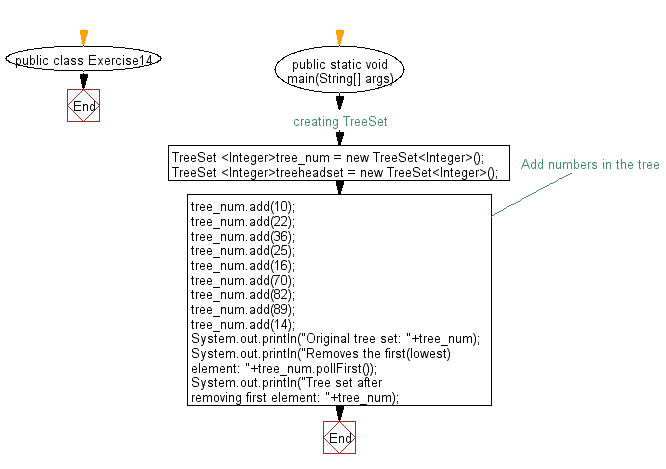﻿ Java Collection, TreeSet Exercises: Retrieve and remove the first element of a tree set - w3resource# Java Collection, TreeSet Exercises: Retrieve and remove the first element of a tree set

## Java Collection, TreeSet Exercises: Exercise-14 with Solution

Write a Java program to retrieve and remove the first element of a tree set.

Sample Solution:

Java Code:

``````import java.util.TreeSet;
import java.util.Iterator;

public class Exercise14 {
public static void main(String[] args) {
// creating TreeSet
TreeSet <Integer>tree_num = new TreeSet<Integer>();
TreeSet <Integer>treeheadset = new TreeSet<Integer>();

// Add numbers in the tree
tree_num.add(10);
tree_num.add(22);
tree_num.add(36);
tree_num.add(25);
tree_num.add(16);
tree_num.add(70);
tree_num.add(82);
tree_num.add(89);
tree_num.add(14);
System.out.println("Original tree set: "+tree_num);
System.out.println("Removes the first(lowest) element: "+tree_num.pollFirst());
System.out.println("Tree set after removing first element: "+tree_num);
}
}
``````

Sample Output:

```Original tree set: [10, 14, 16, 22, 25, 36, 70, 82, 89]
Removes the first(lowest) element: 10
Tree set after removing first element: [14, 16, 22, 25, 36, 70, 82, 89]```

Flowchart:Java Code Editor:

Contribute your code and comments through Disqus.

What is the difficulty level of this exercise?

﻿
+